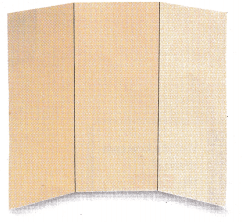Refer to our Texas Go Math Grade 8 Answer Key Pdf to score good marks in the exams. Test yourself by practicing the problems from Texas Go Math Grade 8 Module 16 Answer Key Managing Your Money and Planning for Your Future.

Multiply

Question 1.Explanation:
There are 2 total decimal places in both numbers.
Ignore the decimal places and complete the multiplication as if operating on two integers.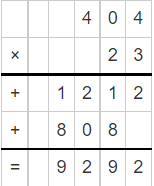Rewrite the product with 2 total decimal places.

Question 2.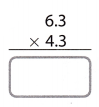Explanation:
There are 2 total decimal places in both numbers.
Ignore the decimal places and complete the multiplication as if operating on two integers.Rewrite the product with 2 total decimal places.

Question 3.Explanation:
There are 2 total decimal places in both numbers.
Ignore the decimal places and complete the multiplication as if operating on two integers.Rewrite the product with 2 total decimal places.

Question 4.Explanation:
There are 4 total decimal places in both numbers.
Ignore the decimal places and complete the multiplication as if operating on two integers.Rewrite the product with 2 total decimal places.

Find the percent.

Question 5.
4% of 40 __________
Explanation:
Finding the fraction of a number is similar to multiplying the fractions with the whole number.
4% = 4/100 or 0.04
4/100 × 40 = 16/10 = 1.6
or
0.04 × 40 = 1.6

Question 6.
7% of 300 ________
Explanation:
Finding the fraction of a number is similar to multiplying the fractions with the whole number.
7% = 7/100 or 0.07
7/100 × 300 = 21
or
0.07 × 300 = 21

Question 7.
4.3% of 1,200 _______
Explanation:
Finding the fraction of a number is similar to multiplying the fractions with the whole number.
4.3% = 4.3/100 or 0.043
4.3/100 × 1200 = 51.6
or
0.043 × 1200 = 51.6

Evaluate. Round to the nearest hundredth.

Question 8.
120(1 + 0.02)2 ____________
Given,
120(1 + 0.02)2
First add the whole number with the decimal number.
120 (1.02)² = 120 × 1.04
120 × 1.04 = 124.8
Now we have to Round to the nearest hundredth.
124.80 to the nearest hundredth.
To round 124.80 to the nearest hundredth consider the thousandths’ value of 124.8, which is 0 and less than 5. Therefore, the hundredths’ value of 124.8 remains 0.

Question 9.
450(1 + 0.05)2 ____________
Given,
450(1 + 0.05)2
First, add the whole number with the decimal number.
450 (1.05)2
450 × 1.1025 = 496.125
Now we have to Round to the nearest hundredth.
496.125 to the nearest hundredth.
To round 496.125 to the nearest hundredth consider the thousandths’ value of 496.125, which is 5 and equal or more than 5. Therefore, the hundredths value of 496.125 increases by 1 to 3.
496.125 to the nearest hundredth is 496.13

Question 10.
900(1 + 0.03)2 ___________
Given,
900(1 + 0.03)2
First, add the whole number with the decimal number.
900(1.03)² = 900 × 1.0609
900 × 1.0609 = 954.81
Now we have to Round to the nearest hundredth.
954.81 to the nearest hundredth.
954.81 to the nearest hundredth is 954.80

Question 11.
75(1 + 0.01)2 ____________
Given,
75(1 + 0.01)2
First, add the whole number with the decimal number.
75(1.01)² = 75 × 1.0201
75 × 1.0201 = 76.50
Now we have to Round to the nearest hundredth.
76.50 to the nearest hundredth is 76.50

Visualize Vocabulary

Use the ✓ words to complete the graphic organizer. You will put one word in each box.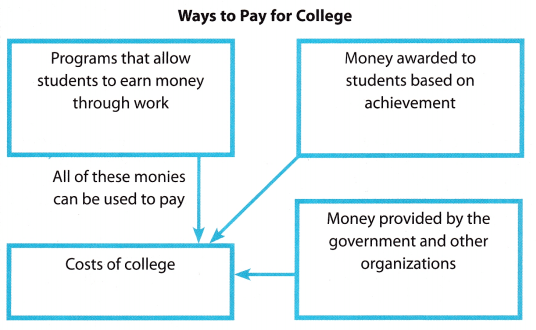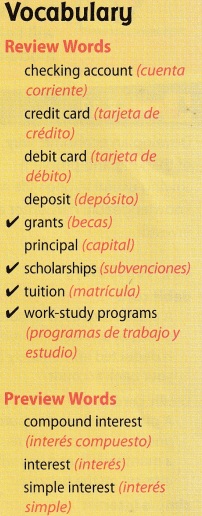Understand Vocabulary

Complete the sentences using the preview words.

Question 1.
The amount of money paid by banks and others to use money in an account is ______________.
Answer: The amount of money paid by banks and others to use money in an account is a checking account.

Question 2.
____________________ is earned on an annual basis using the formula l = Prt.
Answer: Simple Interest is earned on an annual basis using the formula l = Prt.
Interest earned according to this formula is called simple interest.